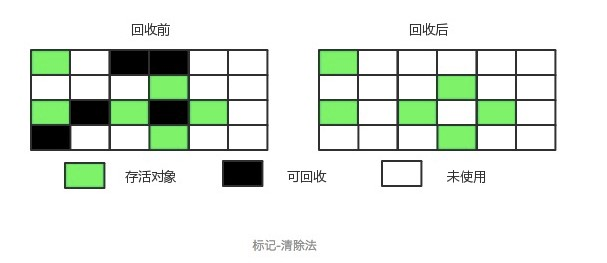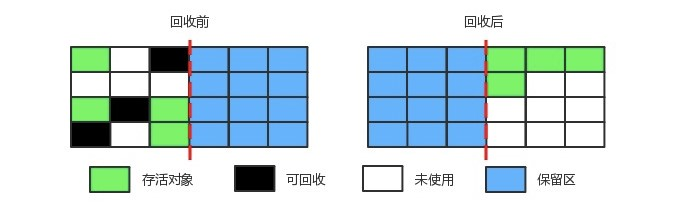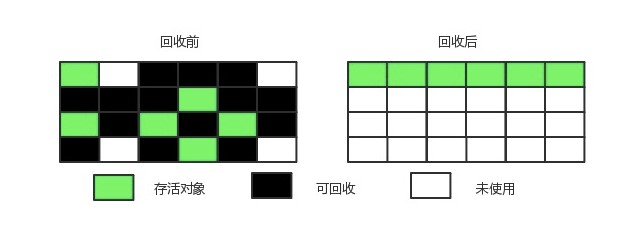# 一、Java

## hashmap

HashMap是常用的Java集合之一，是基于哈希表的Map接口的实现。不支持同步和允许null作为key和value。

HashMap非线程安全，即任一时刻可以有多个线程同时写HashMap，可能会导致数据的不一致。

``````    /**
* 根据key的哈希值和key获取对应的节点
* getNode可分为以下几个步骤：
* 1.如果哈希表为空，或key对应的桶为空，返回null
* 2.如果桶中的第一个节点就和指定参数hash和key匹配上了，返回这个节点。
* 3.如果桶中的第一个节点没有匹配上，而且有后续节点
* 3.1如果当前的桶采用红黑树，则调用红黑树的get方法去获取节点
* 3.2如果当前的桶不采用红黑树，即桶中节点结构为链式结构，遍历链表，直到key匹配
* 4.找到节点返回null，否则返回null。
*/

/**
* putVal方法可以分为下面的几个步骤:
* 1.如果哈希表为空，调用resize()创建一个哈希表。
* 2.如果指定参数hash在表中没有对应的桶，即为没有碰撞，直接将键值对插入到哈希表中即可。
* 3.如果有碰撞，遍历桶，找到key映射的节点
* 3.1桶中的第一个节点就匹配了，将桶中的第一个节点记录起来。
* 3.2如果桶中的第一个节点没有匹配，且桶中结构为红黑树，则调用红黑树对应的方法插入键值对。
* 3.3如果不是红黑树，那么就肯定是链表。遍历链表，如果找到了key映射的节点，就记录这个节点，退出循环。如果没有找到，在链表尾部插入节点。插入后，如果链的长度大于TREEIFY_THRESHOLD这个临界值，则使用treeifyBin方法把链表转为红黑树。
* 4.如果找到了key映射的节点，且节点不为null
* 4.1记录节点的vlaue。
* 4.2如果参数onlyIfAbsent为false，或者oldValue为null，替换value，否则不替换。
* 4.3返回记录下来的节点的value。
* 5.如果没有找到key映射的节点（2、3步中讲了，这种情况会插入到hashMap中），插入节点后size会加1，这时要检查size是否大于临界值threshold，如果大于会使用resize方法进行扩容。
*/

/**
* 对table进行初始化或者扩容。
* 如果table为null，则对table进行初始化
* 如果对table扩容，因为每次扩容都是翻倍，与原来计算（n-1）&hash的结果相比，节点要么就在原来的位置，要么就被分配到“原位置+旧容量”这个位置
* resize的步骤总结为:
* 1.计算扩容后的容量，临界值。
* 2.将hashMap的临界值修改为扩容后的临界值
* 3.根据扩容后的容量新建数组，然后将hashMap的table的引用指向新数组。
* 4.将旧数组的元素复制到table中。
*/
``````

## list与set比较

1. list,set都是集成Collection接口
2. List的特点：元素有放入顺序
3. Set特点：元素无放入顺序，元素不可重复，重复元素会覆盖掉

## volatile原理

volatile是变量修饰符，其修饰的变量具有可见性，Java的做法是将该变量的操作放在寄存器或者CPU缓存上进行，之后才会同步到主存，使用volatile修饰符的变量是直接读写主存，volatile不保证原子性，同时volatile禁止指令重排。

# 二、JVM

## Java内存模型Java虚拟机栈:每个 Java 方法在执行的同时会创建一个栈帧用于存储局部变量表、操作数栈、常量池引用等信息。每一个方法从调用直至执行完成的过程，就对应着一个栈帧在 Java 虚拟机栈中入栈和出栈的过程。

Java堆:几乎所有对象实例都在这里分配内存。是垃圾收集的主要区域（"GC 堆"），虚拟机把 Java 堆分成以下三块：

• 新生代
• 老年代
• 永久代

## 四种引用

``````Object obj = new Object();
``````

``````Object obj = new Object();
SoftReference<Object> sf = new SoftReference<Object>(obj);
``````

``````Object obj = new Object();
WeakReference<Object> wf = new WeakReference<Object>(obj);
``````

``````Object obj = new Object();
PhantomReference<Object> pf = new PhantomReference<Object>(obj);
``````

## gc# 三、数据结构与算法

## 二分查找

``````public class BinarySearch {
public static int binarySearch(int[] array, int key) {
int start = 0;
int end = array.length - 1;
while (start <= end) {
int mid = (end - start) / 2 + start;
if (key < array[mid]) {
end = mid - 1;
} else if (key > array[mid]) {
start = mid + 1;
} else {
return mid;
}
}
return -1;
}

public static int binarySearch_2(int[] array, int start, int end, int key) {
int mid = (end - start) / 2 + start;
if (array[mid] == key) {
return mid;
}
if (start >= end) {
return -1;
} else if (key > array[mid]) {
return binarySearch_2(array, mid + 1, end, key);
} else if (key < array[mid]) {
return binarySearch_2(array, start, mid - 1, key);
}
return -1;
}
}

``````

## 链表反转

``````    public ListNode ReverseList(ListNode head) {
ListNode pre = null;//pre为当前节点的前一节点
ListNode next = null;//next为当前节点的下一节点
//即pre让节点可以反转所指方向，但反转之后如果不用next节点保存next1节点的话，此单链表就此断开了
//所以需要用到pre和next两个节点
//1.2.3.4.5
//1<-2<-3 4.5
//做循环，如果当前节点不为空的话，始终执行此循环，此循环的目的就是让当前节点从指向next到指向pre
}
//直接输出pre就是我们想要得到的反转后的链表
return pre;
}
``````

``````    public ListNode ReverseList(ListNode head) {
//如果链表为空或者链表中只有一个元素
//先递归找到到链表的末端结点，从后依次反转整个链表
//再将当前节点设置为后面节点的后续节点
}
``````

## 二叉树前序遍历

``````	public static void preOrder(TreeNode biTree)
{
System.out.println(biTree.value);
TreeNode leftTree = biTree.left;
if(leftTree != null)
{
preOrder(leftTree);
}
TreeNode rightTree = biTree.right;
if(rightTree != null)
{
preOrder(rightTree);
}
}

public static void preOrder_2(TreeNode biTree)
{
Stack<TreeNode> stack = new Stack<TreeNode>();
while(biTree != null || !stack.isEmpty())
{
while(biTree != null)
{
System.out.println(biTree.value);
stack.push(biTree);
biTree = biTree.left;
}
if(!stack.isEmpty())
{
biTree = stack.pop();
biTree = biTree.right;
}
}
}

``````

## 两个栈实现队列

``````import java.util.Stack;

/**
* 思路：
* 栈A用来作入队列，栈B用来出队列
* 当栈B为空时，栈A全部出栈到栈B,栈B再出栈（即出队列）
*/
public class Solution18 {

Stack<Integer> stack1 = new Stack<Integer>();
Stack<Integer> stack2 = new Stack<Integer>();

public void push(int node) {
stack1.push(node);//stack1负责入队
}

public int pop() {
if (stack1.empty() && stack2.empty()) {
throw new RuntimeException("队列为空");
}
if (stack2.empty()) {
while (!stack1.empty()) {
stack2.push(stack1.pop());
}
}
return stack2.pop();//stcak2负责出队
}
}
``````

## 返回数组中所有出现两次以上的数

``````    /**
* 直接利用hashmap记录出现次数
*
* @param nums
* @return
*/
public List<Integer> findDuplicates(int[] nums) {
List<Integer> res = new ArrayList<>();
if (nums == null || nums.length == 0) {
return res;
}
HashMap<Integer, Integer> map = new HashMap<>();
int count;
for (int val :
nums) {
if (!map.containsKey(val)) {
map.put(val, 1);
} else {
count = map.get(val);
map.put(val, ++count);
}
if (map.get(val) == 2) {
}
}
return res;
}

/**
* 因为数组输入的特点 1<=a[i]<=n,则可以把原数组当hash表用 ，因为原数组是正数，标为负数表示出现过，如果遇到负数就表示第二次出现，就可以找出所有出现过两次的元素
*
* @param nums
* @return
*/
public List<Integer> findDuplicates_2(int[] nums) {
List<Integer> res = new ArrayList<>();
for (int i = 0; i < nums.length; ++i) {
int index = Math.abs(nums[i]) - 1;
if (nums[index] < 0) {
}
nums[index] = -nums[index];
}
return res;
}
``````

## 快排

``````    public static void quickSort(int[] array, int _left, int _right) {
int left = _left;//
int right = _right;
int pivot;//基准线
if (left < right) {
pivot = array[left];
while (left != right) {
//从右往左找到比基准线小的数
while (left < right && pivot <= array[right]) {
right--;
}
//将右边比基准线小的数换到左边
array[left] = array[right];
//从左往右找到比基准线大的数
while (left < right && pivot >= array[left]) {
left++;
}
//将左边比基准线大的数换到右边
array[right] = array[left];
}
//此时left和right指向同一位置
array[left] = pivot;
quickSort(array, _left, left - 1);
quickSort(array, left + 1, _right);
}
}
``````

## 输入一个整数，反转输出:比如输入1234，然后输出4321。

``````    public int reverseInt(int x) {
int ans = 0;
while (x != 0) {
ans = ans * 10 + (x % 10);
x /= 10;
}
if (ans < Integer.MIN_VALUE || ans > Integer.MAX_VALUE) {
ans = 0;
}
return ans;
}
``````

## 最大合数的质子分解，比如输入20，输出2,2,5

``````import java.util.Scanner;

public class Main {
/**
* 进行分解质因数
*
* @param number
*/
public static void factor(int number) {
for (int i = 2; i < number; i++) {
if (number % i == 0) {
System.out.print(i + " ");
//判断number/i是不是素数，如果是素数就直接输出
if (isPrime(number / i)) {
System.out.print(number / i + " ");
} else {
factor(number / i);
}
return;
}
}
}

/**
* 判断是不是素数
*
* @param number
* @return
*/
public static boolean isPrime(int number) {
for (int i = 2; i < number; i++) {
if (number % i == 0) {
return false;
}
}
return true;
}
}
``````

## 链表判断是否有环和找入环的节点。

``````import java.util.HashSet;

public class Solution24 {

/**
* 假设x为环前面的路程，a为环入口到相遇点的路程， c为环的长度
* 当快慢指针相遇的时候： 此时慢指针走的路程为Sslow = x + m * c + a
* 快指针走的路程为Sfast = x + n * c + a
* 2 Sslow = Sfast
* 2 * ( x + m*c + a ) = (x + n *c + a)
* 从而可以推导出： x = (n - 2 * m )*c - a = (n - 2 *m -1 )*c + c - a
* 即环前面的路程 = 数个环的长度（为可能为0） + c - a
* 什么是c - a？这是相遇点后，环后面部分的路程。
* 所以，我们可以让一个指针从起点A开始走，让一个指针从相遇点B开始继续往后走， 2个指针速度一样，
* 那么，当从原点的指针走到环入口点的时候（此时刚好走了x） 从相遇点开始走的那个指针也一定刚好到达环入口点。
* 所以两者会相遇，且恰好相遇在环的入口点。
*
* @return
*/
return null;
//先判断有没有环
while (fast != slow) {
if (fast.next != null && fast.next.next != null) {
fast = fast.next.next;
slow = slow.next;
} else {
//没有环
return null;
}
}

//有环
while (fast != slow) {
fast = fast.next;
slow = slow.next;
}
return fast;
}

/**
* 利用HashSet元素不能重复
*
* @return
*/
HashSet<ListNode> hashSet = new HashSet<>();
}
}
return null;
}

public class ListNode {
int val;
ListNode next = null;

ListNode(int val) {
this.val = val;
}
}
}
``````

## 数组中出现次数超过一半的数字

``````import java.util.HashMap;

public class Solution42 {
/**
* 利用 Boyer-Moore Majority Vote Algorithm（摩尔投票算法）来解决这个问题
*
* @param array
* @return
*/
public int MoreThanHalfNum_Solution_2(int[] array) {
int majority = array;
for (int i = 1, count = 1; i < array.length; i++) {
count = array[i] == majority ? count + 1 : count - 1;
if (count == 0) {
majority = array[i];
count = 1;
}
}
int count = 0;
for (int val : array) if (val == majority) count++;
return count > array.length / 2 ? majority : 0;
}

/**
* 利用HashMap记录每个数字以及数字出现的次数
*
* @param array
* @return
*/
public int MoreThanHalfNum_Solution(int[] array) {
if (array == null || array.length == 0)
return 0;
HashMap<Integer, Integer> map = new HashMap<>();
int count;
for (int val :
array) {
if (!map.containsKey(val)) {
map.put(val, 1);
} else {
count = map.get(val);
map.put(val, ++count);
}
if (map.get(val) > array.length / 2) {
return val;
}
}
return 0;
}
}
``````

## 和为S的两个数字

``````import java.util.ArrayList;
import java.util.HashMap;

public class Solution34 {
/**
* 两头夹逼
*
* @param array
* @param sum
* @return
*/
public ArrayList<Integer> FindNumbersWithSum(int[] array, int sum) {
ArrayList<Integer> result = new ArrayList<>();
int left = 0;
int right = array.length - 1;
while (left < right) {
if (array[left] + array[right] == sum) {
break;
} else if (array[left] + array[right] > sum) {
right--;
} else {
left++;
}
}
return result;
}

/**
* 用HashMap存放元素和下标，然后开始遍历数组找到和为sum的两个元素，从左到右找到的第一对和为sum的就是最小的一对。
*
* @param array
* @param sum
* @return
*/
public ArrayList<Integer> FindNumbersWithSum_2(int[] array, int sum) {
HashMap<Integer, Integer> map = new HashMap<>();
ArrayList<Integer> result = new ArrayList<>();
int len = array.length;
for (int i = 0; i < len; i++) {
map.put(array[i], i);
}
for (int i = 0; i < len; i++) {
int sub = sum - array[i];
if (map.containsKey(sub)) {
break;
}
}
return result;
}
}

``````

# 四、操作系统

## 线程&&进程区别，什么情况下多进程要优于多线程

• 进程是资源分配的基本单位，但是线程不拥有资源，线程可以访问隶属进程的资源。
• 线程是独立调度的基本单位，在同一进程中，线程的切换不会引起进程切换，从一个进程内的线程切换到另一个进程中的线程时，会引起进程切换。
• 由于创建或撤销进程时，系统都要为之分配或回收资源，如内存空间、I/O 设备等，所付出的开销远大于创建或撤销线程时的开销。类似地，在进行进程切换时，涉及当前执行进程 CPU 环境的保存及新调度进程 CPU 环境的设置，而线程切换时只需保存和设置少量寄存器内容，开销很小。
• 进程间通信需要进程同步和互斥手段的辅助，以保证数据的一致性。而线程间可以通过直接读/写同一进程中的数据段（如全局变量）来进行通信（需要做好同步）。

• 不需要频繁创建销毁的优先用线程
• 不需要进行大量计算的优先使用线程
• 弱相关的处理用进程
• 可能要扩展到多机分布的用进程

1. 进程管理

1. 内存管理

1. 文件管理

1. 设备管理

# 五、网络与数据库

## HTTPS

HTTPS(Secure Hypertext Transfer Protocol) 安全超文本传输协议是一个安全的通信通道，它基于HTTP开发，用于在客户计算机和服务器之间交换信息。HTTPS使用安全套接字层(SSL)进行信息交换，简单来说HTTPS是HTTP的安全版，是使用TLS/SSL加密的HTTP协议。

HTTPS 通信过程

• 客户端发送请求到服务器端
• 服务器端返回证书和公开密钥，公开密钥作为证书的一部分而存在
• 客户端验证证书和公开密钥的有效性，如果有效，则生成共享密钥并使用公开密钥加密发送到服务器端
• 服务器端使用私有密钥解密数据，并使用收到的共享密钥加密数据，发送到客户端
• 客户端使用共享密钥解密数据
• SSL加密建立

## 详细问TCP协议

TCP就是单纯建立连接，不涉及任何我们需要请求的实际数据，简单的传输。

## SSL握手

• 客户端发送随机数1，支持的加密方法（如RSA公钥加密）
• 服务端发送随机数2，和服务器公钥，并确认加密方法
• 客户端发送用服务器公钥加密的随机数3
• 服务器用私钥解密这个随机数3，用加密方法计算生成对称加密的密钥给客户端，
• 接下来的报文都用双方协定好的加密方法和密钥，进行加密

## Redis介绍

Redis 是一个高性能的key-value数据库。

Redis 与其他 key - value 缓存产品有以下三个特点：

1. Redis支持数据的持久化，可以将内存中的数据保存在磁盘中，重启的时候可以再次加载进行使用。
2. Redis不仅仅支持简单的key-value类型的数据，同时还提供list，set，zset，hash等数据结构的存储。
3. Redis支持数据的备份，即master-slave模式的数据备份。

## 数据库隔离机制

• 可串行化（SERIALIXABLE）：强制事务串行执行。可避免脏读、不可重复读、幻读的发生。

## redis的基本数据类型

Redis支持五种数据类型：string（字符串），hash（哈希），list（列表），set（集合）及zset(sorted set：有序集合)。

## redis的过期机制

• 在进行get或set等操作时，先检查key是否过期，
• 若过期，删除key，然后执行相应操作；
• 若没过期，直接执行相应操作

• 遍历每个数据库（就是redis.conf中配置的"database"数量，默认为16）
• 检查当前库中的指定个数个key（默认是每个库检查20个key，注意相当于该循环执行20次，循环体时下边的描述）
• 如果当前库中没有一个key设置了过期时间，直接执行下一个库的遍历
• 随机获取一个设置了过期时间的key，检查该key是否过期，如果过期，删除key
• 判断定期删除操作是否已经达到指定时长，若已经达到，直接退出定期删除。

## servlet的生命周期

Servlet有三个生命周期函数，初始化方法init(),处理客户请求的方法service(),终止方法destroy()。

# 六、设计模式

## 单例

``````public class Singleton {
private volatile static Singleton instance = null;

private Singleton() {

}

/**
* 当第一次调用getInstance()方法时，instance为空，同步操作，保证多线程实例唯一
* 当第一次后调用getInstance()方法时，instance不为空，不进入同步代码块，减少了不必要的同步
*/
public static Singleton getInstance() {
if (instance == null) {
synchronized (Singleton.class) {
if (instance == null) {
instance = new Singleton();
}
}
}
return instance;
}
}
``````

# 七、框架

## spring特性

IOC:控制反转也叫依赖注入，IOC利用java反射机制。所谓控制反转是指，本来被调用者的实例是有调用者来创建的，这样的缺点是耦合性太强，IOC则是统一交给spring来管理创建，将对象交给容器管理，你只需要在spring配置文件总配置相应的bean，以及设置相关的属性，让spring容器来生成类的实例对象以及管理对象。在spring容器启动的时候，spring会把你在配置文件中配置的bean都初始化好，然后在你需要调用的时候，就把它已经初始化好的那些bean分配给你需要调用这些bean的类。

AOP是对OOP的补充和完善。AOP利用的是代理，分为CGLIB动态代理和JDK动态代理。OOP引入封装、继承和多态性等概念来建立一种对象层次结构。OOP编程中，会有大量的重复代码。而AOP则是将这些与业务无关的重复代码抽取出来，然后再嵌入到业务代码当中。实现AOP的技术，主要分为两大类：一是采用动态代理技术，利用截取消息的方式，对该消息进行装饰，以取代原有对象行为的执行；二是采用静态织入的方式，引入特定的语法创建“方面”，从而使得编译器可以在编译期间织入有关“方面”的代码，属于静态代理。

## mybatis中，配置sql,propertyType 有一个int类型，一个String类型，怎么配置

hashmap，把这两个放到map中